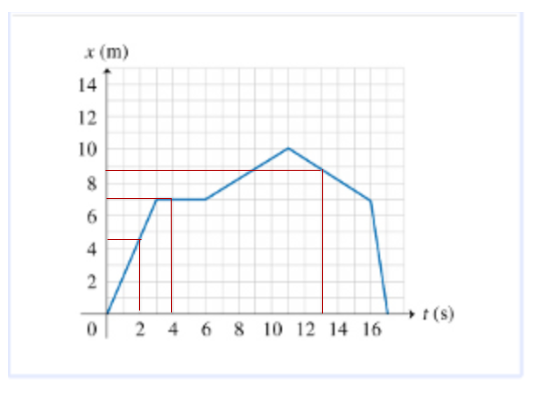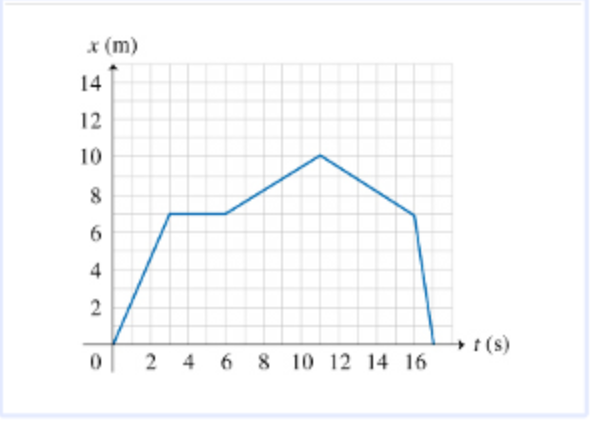# Problem: Part A Find the object's positions x1, x2, x3, and x4 at times t1 = 2.0 s, t2 = 4.0 s, t3 = 13 s, and t4 = 17 s. Express your answer in meters to one significant figures, separated by commas. Part BFind the object's speeds v1, v2, and v3 at times t1 = 2.0 s, t2 = 4.0 s, and t3 = 13 s.Express your answers in meters per second to two significant figures, separated by commas.

###### FREE Expert Solution

Part AVertical scale, 1 square = 1 m

x1 = 4.5 × 1 m = 4.5 m

x2 = 7 × 1 m = 7 m

x3 = 8.75 × 1 m = 8.8 m

x4 = 0 m

98% (425 ratings)###### Problem DetailsPart A

Find the object's positions x1, x2, x3, and x4 at times t1 = 2.0 s, t2 = 4.0 s, t3 = 13 s, and t4 = 17 s.

Express your answer in meters to one significant figures, separated by commas.

Part B

Find the object's speeds v1, v2, and v3 at times t1 = 2.0 s, t2 = 4.0 s, and t3 = 13 s.

Express your answers in meters per second to two significant figures, separated by commas.